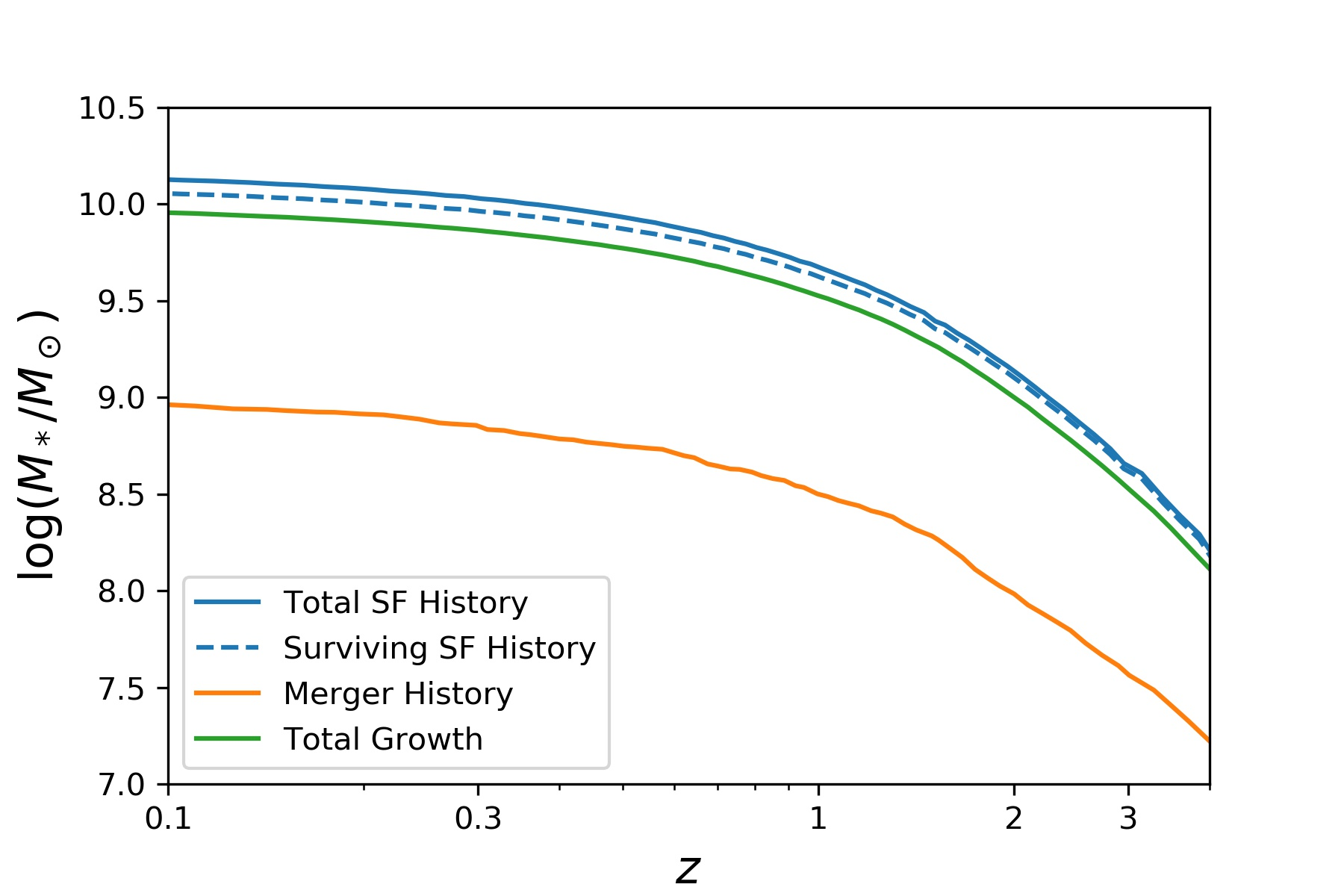### Surving star formation historiesMax Dickson
• 26 Apr

I am attempting to get the mean surviving star formation histories of galaxies within bins of stellar mass at z=0. To do this I am extracting the SFRs from each galaxy's tree and calculating the mean SFR at each snapshot. I then correct the SFR for mass lost to the interstellar medium using a functional form of Global Mass Loss Rate (GMLR) (Leitner and Kravstov 2011 (https://arxiv.org/pdf/1011.1252.pdf) if you are interested), which is based on the SFR and two parameters derived from the Initial Mass Function (IMF). Specifically, the parameters are based on the fraction of stars that have masses greater than the main sequence turn-off mass, as defined by the IMF. I then integrate SFR(t) - GMLR(t) over t to find the mean surviving star formation histories.

I have tried using the fitting parameters for the GMLR derived from a Cabrier 2003 IMF (provided by the aforementioned paper), however, this yields a surviving SFH which is greater than the total growth of the galaxies, as shown in the attached image.My intention is to compare the sum of the merger contribution and surviving SFH to the total growth, which only makes sense if the surviving SFH is lower than the total growth. Is there a particular reason why this sort of methodology would not work with TNG?

Thanks!Dylan Nelson
• 26 Apr

Can you clarify what "mean surviving star formation history" is? Is this the SFH of the stars which make up the galaxy at z=0?

Two quick notes:

(1) An alternative way to get SFHs, instead of using the SFR(t) along the merger tree, is to histogram the ages (i.e. age of the universe at the time of birth, derived from `PartType4/GFM_StellarFormationTime`) of all star particles belonging to a galaxy. You can see this has a slightly different meaning.

(2) While such a functional form for mass loss should be approximately right, it won't exactly represent the mass loss used in the TNG model. However, we have an exact record of the amount of mass loss for every star particle (the difference of `PartType4/Masses` and `PartType4/GFM_InitialMass`), so you can compute the actual mass loss for any star or galaxy, if needed.Max Dickson
• 1 May

Hi Dylan,

Yes, that is what I mean by "surviving star formation history", specifically the amount of stellar mass still present at each redshift that was formed within the galaxy (ie not acquired ex-situ). I took a slightly different approach to what you suggested and fitted the parameters of the "fraction of mass loss compared to the initial mass" formula from the aforementioned paper (eq 1) to the data from the catalogs you suggested for 100 galaxies.

Curiously, the mass loss rate was actually lower than the mass loss rate predicted by the Chabrier 2003 IMF, resulting in a very similar plot to the one I showed before. This would imply that there is some other mechanism causing galaxies to consistently lose stellar mass (or, perhaps more likely, my methodology is wrong). Is this a possibility?

Thanks!

• Page 1 of 1